# RF Oscillator Uses Current-Feedback Op Amp

## Texas Instruments LM6181

A current-feedback amplifier is a well-known component with many uses. Its basic block diagram shows that its input stage is a voltage follower – in practice, a symmetrical emitter follower (Figure 1). The configuration samples the output current, converts it to voltage across a large impedance, and amplifies it to the output using a high-power, low-output-impedance amplifier. The idea is to use the amplifier's input stage as a voltage follower in a basic Colpitts oscillator. This circuit uses the noninverting input of the current-feedback amplifier as the follower input and the inverting input of the amplifier as the follower output. You use the output amplifier to obtain a relatively high-power buffered output. The circuit in Figure 2 shows a basic Colpitts oscillator that uses the amplifier's input-voltage follower as the active element of the oscillator.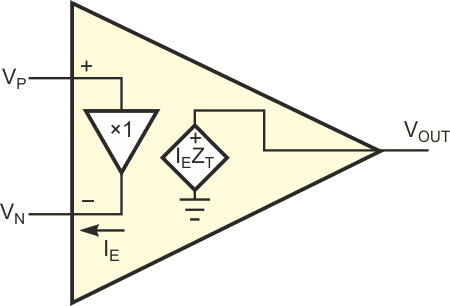Figure 1. In a typical current feedback amplifier, the input stage is a voltage follower.

Take note of two aspects of this oscillator circuit: First, back-to-back diodes connect across the resonator to limit the oscillations to a specific level, thus maintaining the linearity of the voltage follower. Second, the voltage follower output connects to the resonator tap through resistor ROSC to improve the linearity and define the feedback magnitude. The value of ROSC, 330 Ω, lets you obtain soft clipping operation of the diodes across the resonator (VRES = 1 V p-p, which is 0.5 V peak across each diode). Figure 3 shows VRES, the measured voltage at the top of the resonator. RF is the amplifier's feedback resistor; the amplifier's manufacturer recommends its value. This design uses the LM6181, and the value of RF is 1 kΩ.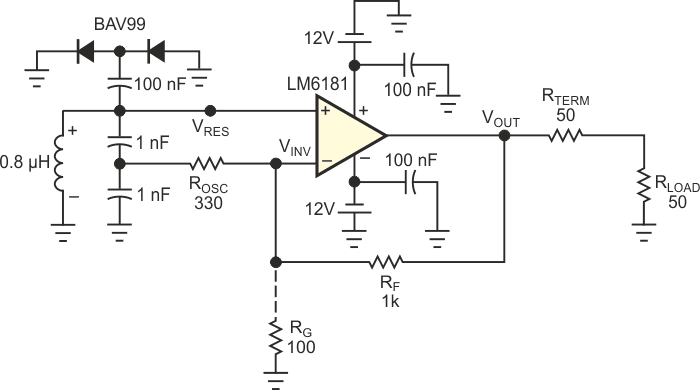Figure 2. This Colpitts oscillator uses a current-feedback amplifier to provide a clean sinusoidal output.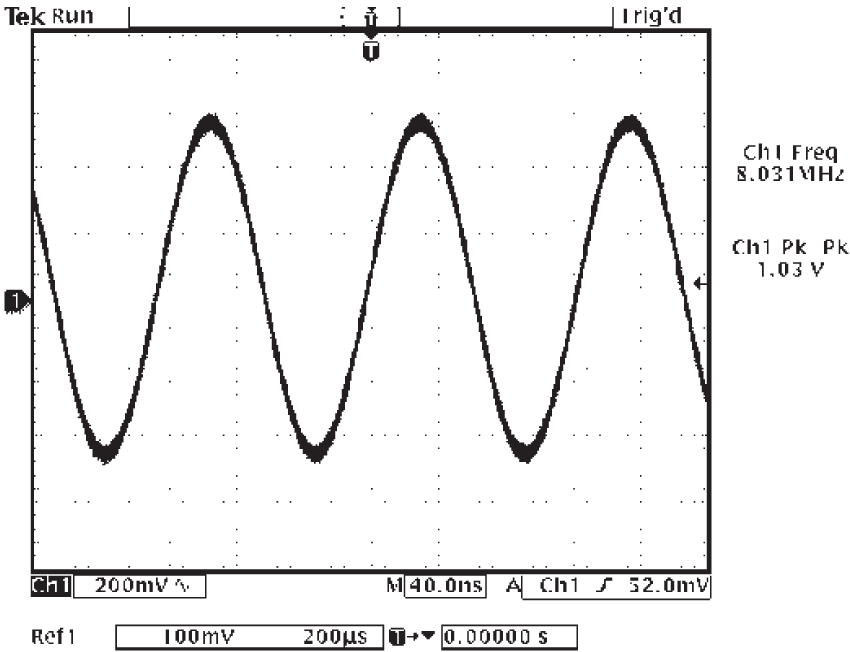Figure 3. This clean sinusoid is the signal at the top of the resonator, VRES, in Figure 2.

It is easy to calculate the output voltage:

VRES = 1 V p-p,

and

VINV = VRES = 1 V p-p.

The voltage-buffer gain is unity: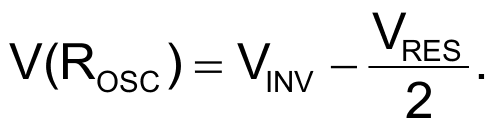The voltage at the resonator tap is VRES/2, because the resonator capacitors are equal in value.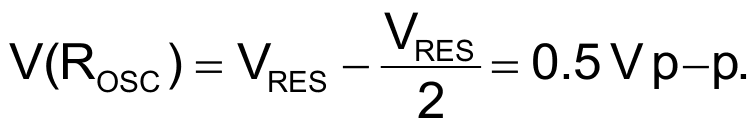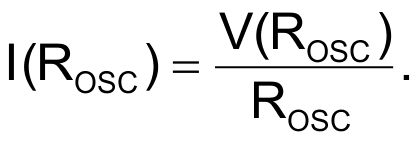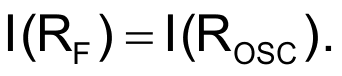The negative feedback nulls the amplifier's inverting-input current.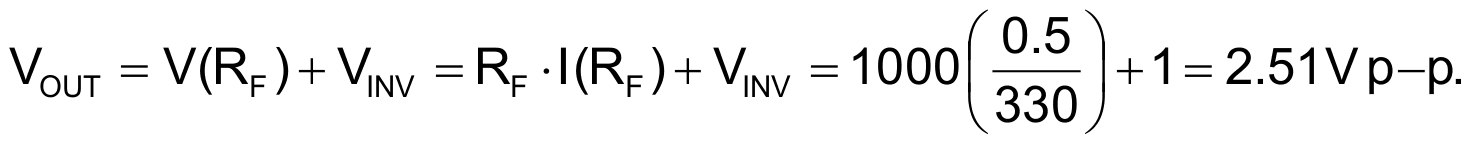If you need more voltage, you can add RG – in this case, 100 Ω – from the inverting input to ground.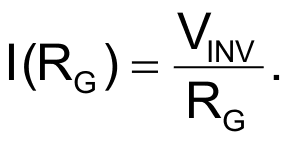Now, the current through RF is the sum of the currents through ROSC and RG. So,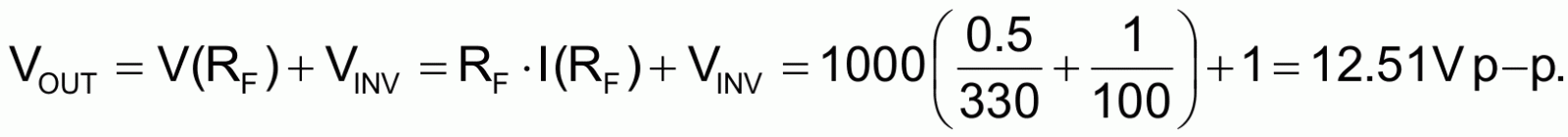Figure 4 shows the measured output voltage.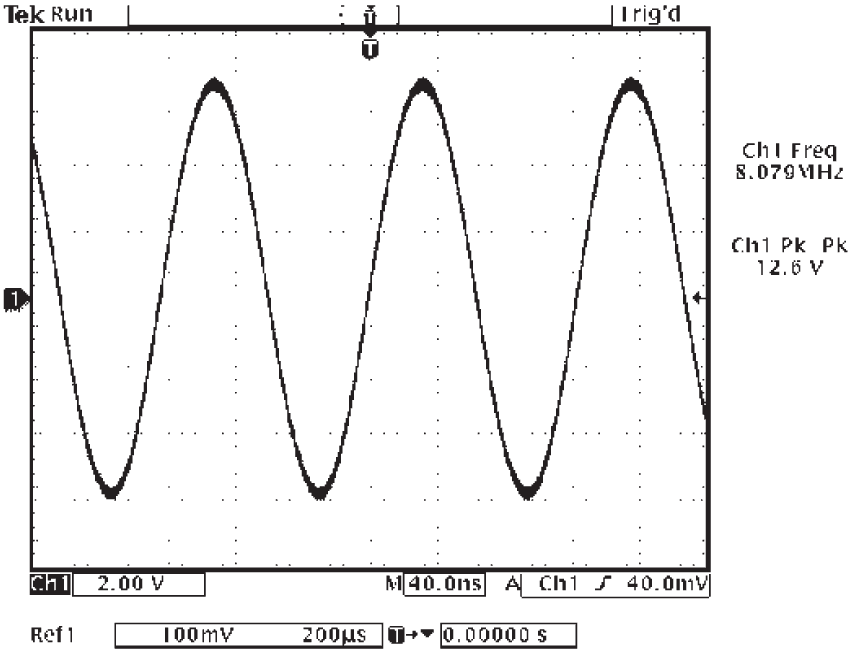Figure 4. The Colpitts oscillator in Figure 2 produces a pure sinusoidal output.

The LM6181's maximum output current is 100 mA, so it can easily drive a current of ±63 mA p-p (±6.3 V/100 Ω) into a total load of 100 Ω (50 Ω output-termination resistor and 50 Ω load resistor). The voltage across the 50 Ω load is 3.15 V peak, or 2.23 V rms, which is close to 20 dBm (100 mW). This power level can directly drive high-level diode double-balanced mixers, or it can drive a higher power amplifier while delivering a clean sinusoidal waveform. You can modify the resonator circuit to accommodate different tuning elements. You can use the circuit as a crystal oscillator by changing the inductor to a crystal and changing the resonator capacitors to an appropriate value, such as 2 × 68 pF. You need a high-value, such as 10-kΩ, bias resistor from the noninverting input to ground to provide bias current to this input.

## Materials on the topic

EDN